Author: Oscar Cronquist Article last updated on June 20, 2018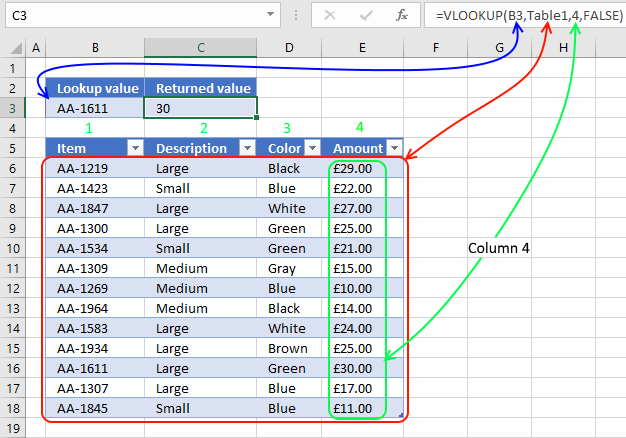The VLOOKUP function lets you search the leftmost column for a value and return another value on the same row in a column you specify.

VLOOKUP stands for vertical lookup and your data (table_array) must be organized into records, a record on each row. To search records excel must look vertically in the data array. HLOOKUP stands for horizontal lookup but that is for another post.

Formula in cell C3:

=VLOOKUP(B3,B6:E18,4,FALSE)

The VLOOKUP function uses lookup value AA-1611 in cell B3 and searches Table1 (cell range B6:E18) in the leftmost column. A matching value is found on row 16.

The third argument is which column you want to fetch the corresponding value from. In this example column 4 is entered and £30.00 is returned in cell C3.

The fourth and last argument lets you choose between approximate and exact match, in this case FALSE meaning EXACT match.

### Excel function syntax

VLOOKUP(lookup_value, table_array, col_index_num, [range_lookup])

### Arguments

lookup_value - Value you want to look up

table_array - The range you want to use, remember that the VLOOKUP function always looks in the leftmost column in your specified range.

col_index_num - The column number which contains the return value

[range_lookup] - True or False (boolean value). True - approximate match, leftmost column must be sorted ascending. False - Exact match

#### Approximate match

The fourth argument allows you to choose if you want APPROXIMATE or EXACT match. You want, in most cases, to use the EXACT match however the default value is APPROXIMATE match. So make sure you know what you are doing.

This example below shows you the difference between EXACT and APPROXIMATE match.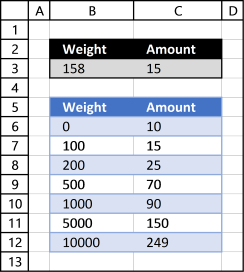You must have your leftmost column sorted in an ascending order or you may get incorrect results.

Approximate match is useful if you want to find which group or range your value belongs to. The example above shows that value 158 is between 100 and 200 so the return value is 15.

Formula in cell C3:

=VLOOKUP(B3,B6:C12,2,TRUE)

A pivot table can't work with related tables (power pivots do) however the VLOOKUP function can quickly merge related tables so you then can analyze data in a pivot table.

These two tables share a data column (invoice), you can use the following formula to merge these two tables.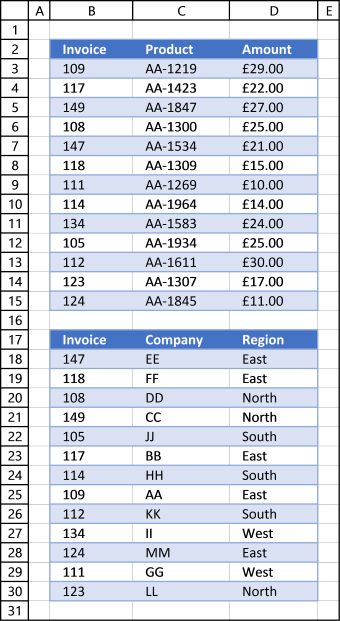Formula in cell E3:

=VLOOKUP(\$B3, \$B\$18:\$D\$30, COLUMN(B2),FALSE)

Copy formula in cell E3 and paste to E3:F15.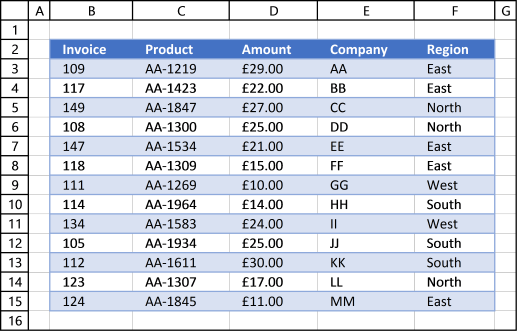#### Dynamic arguments

This example shows you how to search multiple tables. The image below shows two excel tables, table10 and table20.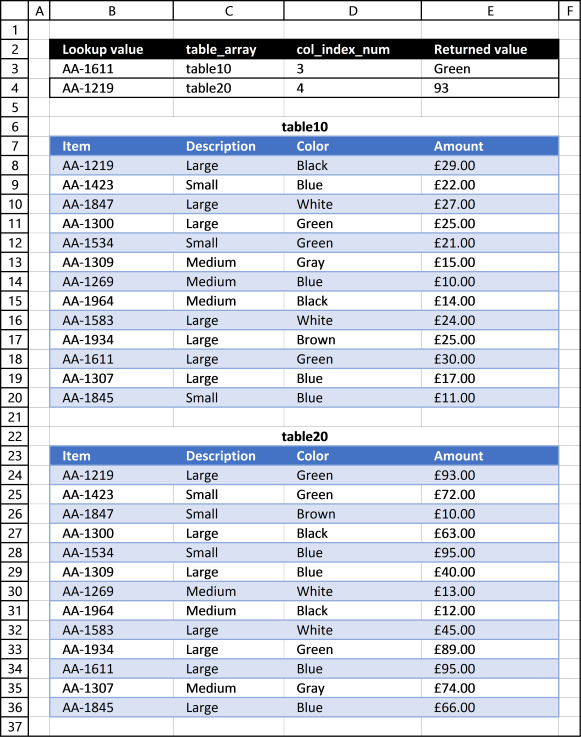Formula in cell E3:

=VLOOKUP(B3,INDIRECT(C3),D3,FALSE)

The INDIRECT function lets you use a cell value as the table_array argument, allowing you to search multiple tables.

#### INDEX + MATCH

The VLOOKUP function is that it can only look for a value in the leftmost column. If you don't have your values in the leftmost column you have two options, rearrange your table or use another method, shown below.The INDEX and MATCH function allows you to search any column in a table and return a value in any column on the same row. This formula is so versatile that I actually prefer INDEX + MATCH over the VLOOKUP function.

Formula in cell C3:

=INDEX(G6:G18,MATCH(D3,E6:E18,0))

The MATCH function lets you look for a value in a column and return the relative position of the found value in the array. AA-1611 is found on row 11 in cell range C6:C18

#### VLOOKUP Error

The IFERROR function allows you to return a value if the VLOOKUP function returns an error, in this case NOT FOUND!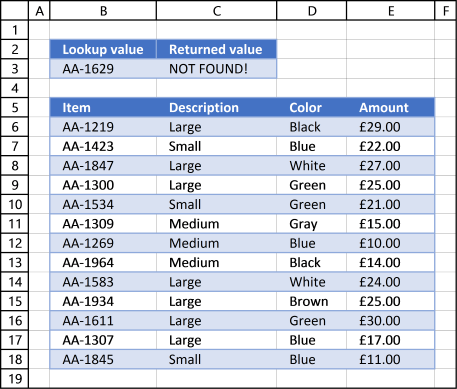#### VBA Example

The following VBA code demonstrates how to use VLOOKUP in a macro.The macro searches B6:B18 using the the value in cell C2 and displays the result in a messagebox.

```VBA code
Sub VLP()
MsgBox Application.WorksheetFunction.VLookup(Range("C2"), Range("B6:E18"), 4, False)
End Sub
```